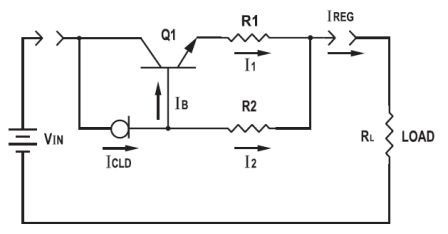# Practical Circuit Design Technique in Boosting Current Limit of CLDs by Using Transistor Current Gain

Practical Circuit Design Technique in Boosting Current Limit of CLDs by Using Transistor Current Gain - If you find that such an impractical way by juPractical Circuit Design Technique in Boosting Current Limit of CLDs by Using Transistor Current Gain - If you find that such an impractical way by just adding the Current Limiting Diodes (CLD) to obtain high current level,

Current Booster with NPN transistor Circuit Diagramthe following article may help you regarding the informations within.

This article will describe you with a simplified description of practical circuit design technique in boosting current limit by using transistor current gain.

What you will find in this aplication datasheet article are sections that discuss about the practical current booster circuit technique (conventional circuit using CLD, current boosting circuit technique),

the analysis of booster circuit principle, the implementation of circuit considerations, and an exemplary experimental result with ilustration using NPN CZT3055/ PNPCZT2955 transistors.

Also you will find current booster circuit diagrams for both NPN and PNP transistors. Finally-according to the article, a booster circuit can be comprised of only one transistor, one CLD, and two resistors.

This circuit can boost the current of CLDs to much higher levels limited only by the current capacity and current gain of the transistor used.

The article was well-written by the respectfull of Sze Chin, Senior Applications Engineer for Central Semiconductor Corp. in Hauppauge, NY.

See complete read of Practical Circuit Design Technique in Boosting Current Limit of CLDs by Using Transistor Current Gain within this application datasheet article (source: centralsemi.com)

Tags: current boosting circuit, CLD, Current Booster, Transistor,

Copyright www.unboxing.eu.org